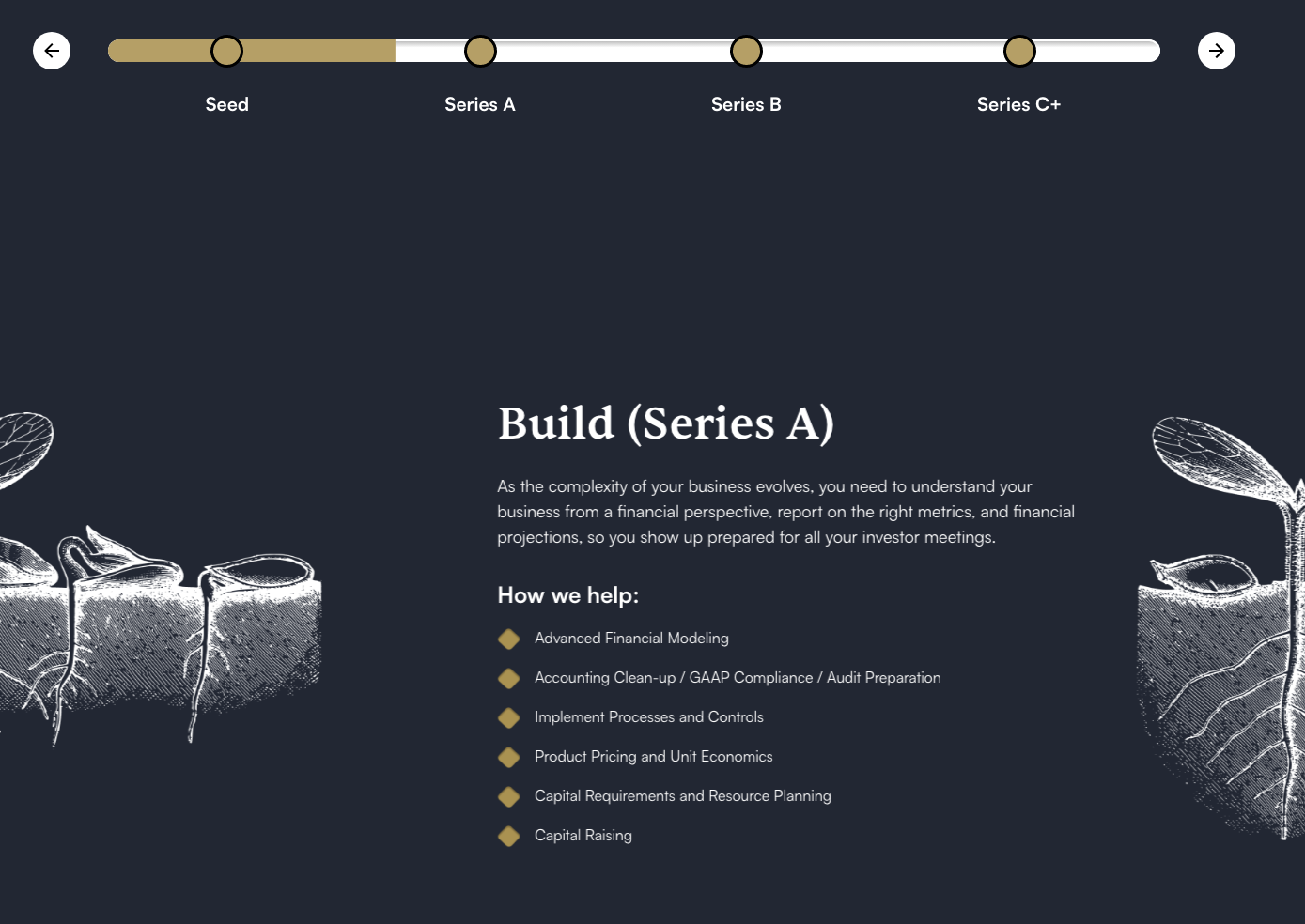## Navigate a horizontal scroll animation in Webflow using buttons

Here is a way to navigate your horizontal scroll animations in Webflow using custom arrow buttons. Enjoy!

(Still under development as of March 15th 2023)My good friend Davor Eyged needed a way to navigate his horizontal scroll animation in Webflow using custom arrow buttons

Here is the code that made this possible: (Have fun and try to make it work for your project)

``````// *** Progress bar code ***

// Define global elements
const array_of_\$_triggers = \$('.progress-bar_circle.hide-tablet')
const \$_left_arrow = \$('.stack2_arrow-cnt').find('.js-prev')
const \$_right_arrow = \$('.stack2_arrow-cnt').find('.js-next')
const \$_slide_wrapper = \$('.stack2-slides-wrapper')
// Define global strings

// Main function
function main() {
\$_left_arrow.click(() => {
scroll_to_slide('left')
})
\$_right_arrow.click(() => {
scroll_to_slide('right')
})

}

// Helper functions
// Scroll to slide
const inaccuracy_buffer_float = 0.055 // = xx.x %
function scroll_to_slide( direction ) {
// Set local variables
var slide_width = \$(window).width()
var slide_wrapper_position = parseInt( \$_slide_wrapper.css('-webkit-transform').split(',') ) * -1
// Calculate
var inaccuracy_buffer = slide_width * inaccuracy_buffer_float
var x = this_slide_is_next( direction, slide_width, inaccuracy_buffer, slide_wrapper_position )

// Scroll to slide x
array_of_\$_triggers[x].click()

}
// This slide is next
function this_slide_is_next( direction, width, buffer, position ) {

// Logic
var min = 0
var max = array_of_\$_triggers.length - 1
var int = 0

if ( direction == 'left' ) {
int = Math.ceil( (position - buffer) / width )

if ( int < min + 1 ) {
int = min
} else {
int -= 1
}

} else if ( direction == 'right' ) {
int = Math.floor( (position + buffer) / width )
if ( int > max - 1 ) {
int = max
} else {
int += 1
}

}
return int

}

// Call main function
main()``````

## FAQs

You still have an open question about BMG.studio and how we operate. Chances are good that you will find what you are looking for here:

### Why does this FAQ exist?

Well. As of Wednesday, March 15th 2023, I think this component looks really cool.

### Can I clone this FAQ component?

Yes. Yes you can. You can do so -> here <-

#### Still have questions?

It's okay to reach out. Use either email or our calendar tool